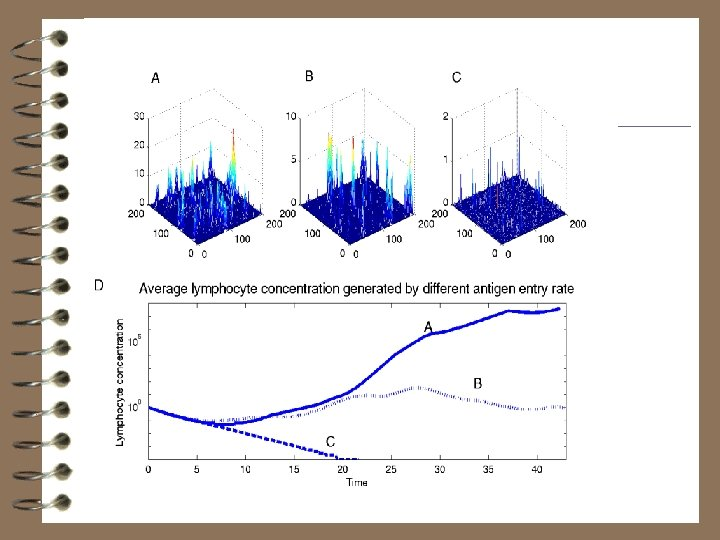# Seminar in mathematical Biology Theoretical issues in modeling

• Slides: 56Seminar in mathematical Biology Theoretical issues in modeling Yoram Louzoun Nadav Shnerb Eldad Bettelheim Sorin Solomon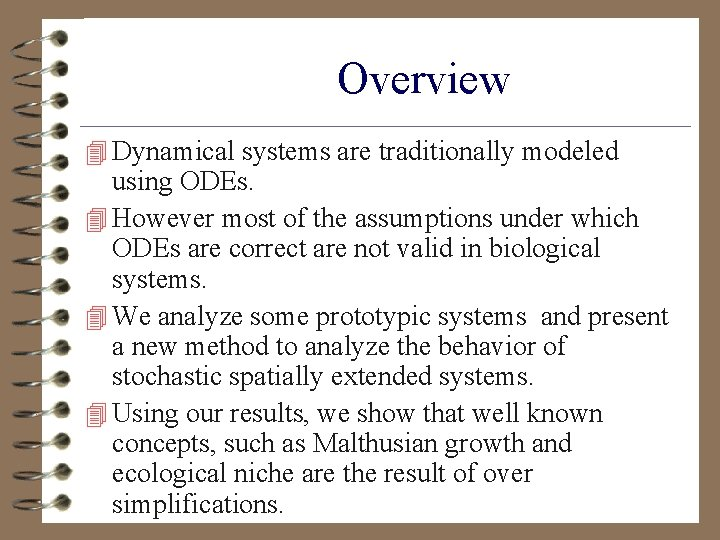Overview 4 Dynamical systems are traditionally modeled using ODEs. 4 However most of the assumptions under which ODEs are correct are not valid in biological systems. 4 We analyze some prototypic systems and present a new method to analyze the behavior of stochastic spatially extended systems. 4 Using our results, we show that well known concepts, such as Malthusian growth and ecological niche are the result of over simplifications.Fisher Equation 4 The simplest system contains a single agent and three processes: Birth, death and competition. 4 Such system is represented by a Fisher Equation. 4 is the birth rate- the death rate, is the competition rate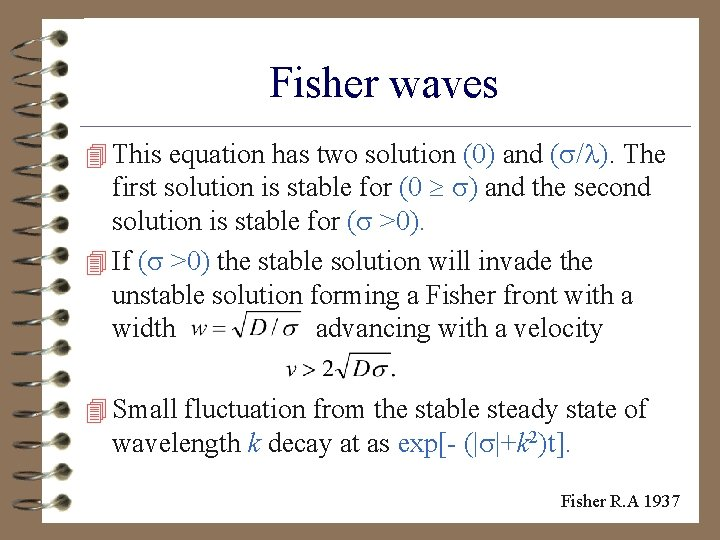Fisher waves 4 This equation has two solution (0) and ( / ). The first solution is stable for (0 ) and the second solution is stable for ( >0). 4 If ( >0) the stable solution will invade the unstable solution forming a Fisher front with a width advancing with a velocity 4 Small fluctuation from the stable steady state of wavelength k decay at as exp[- (| |+k 2)t]. Fisher R. A 1937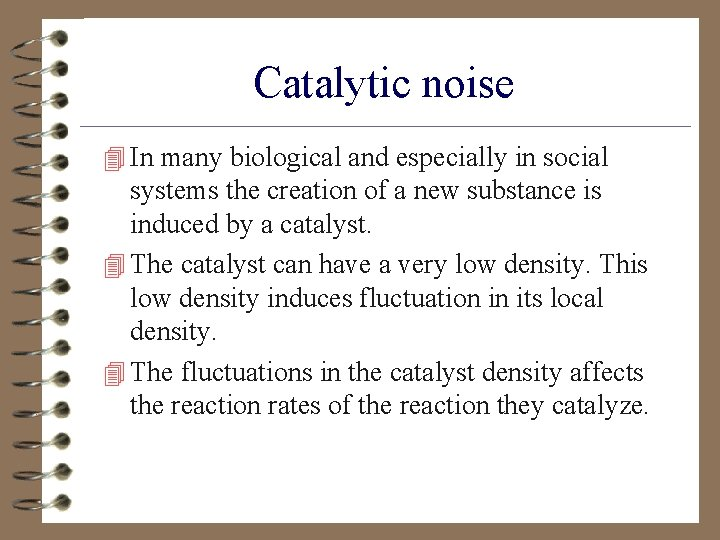Catalytic noise 4 In many biological and especially in social systems the creation of a new substance is induced by a catalyst. 4 The catalyst can have a very low density. This low density induces fluctuation in its local density. 4 The fluctuations in the catalyst density affects the reaction rates of the reaction they catalyze.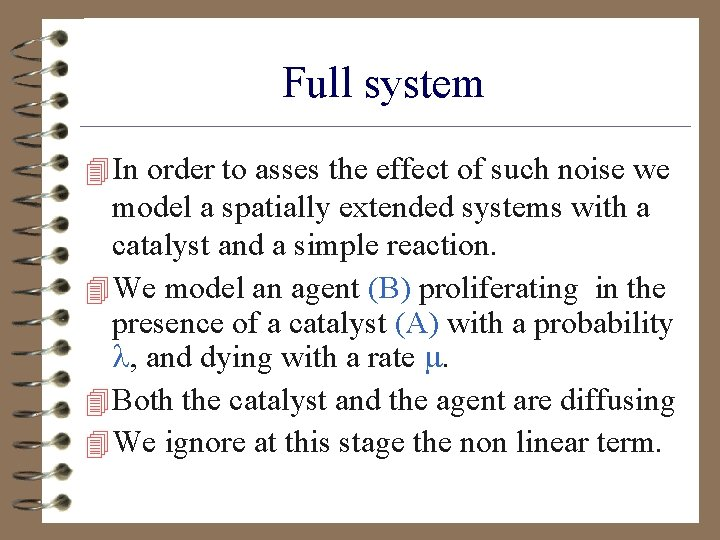Full system 4 In order to asses the effect of such noise we model a spatially extended systems with a catalyst and a simple reaction. 4 We model an agent (B) proliferating in the presence of a catalyst (A) with a probability , and dying with a rate . 4 Both the catalyst and the agent are diffusing 4 We ignore at this stage the non linear term.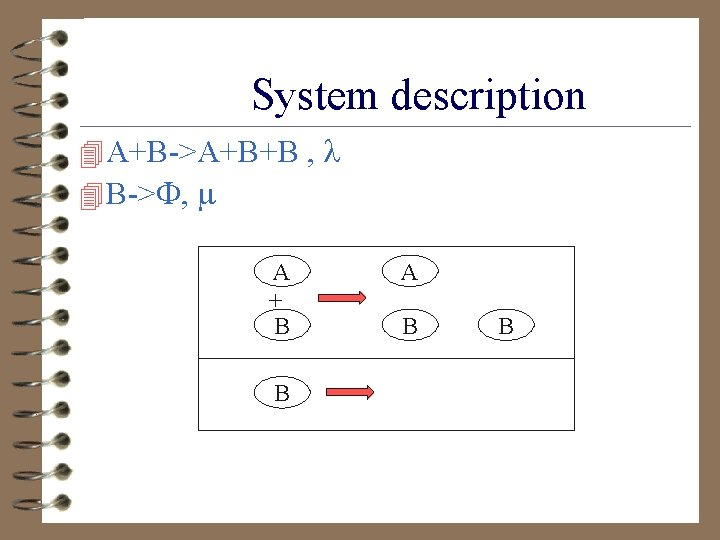System description 4 A+B->A+B+B , 4 B-> , A + B B A B BNaive PDE 4 The PDE describing this system is: 4 If we let the A diffuse for a long time, before B reactions starts the A distribution will be: 4 A(x)=A 0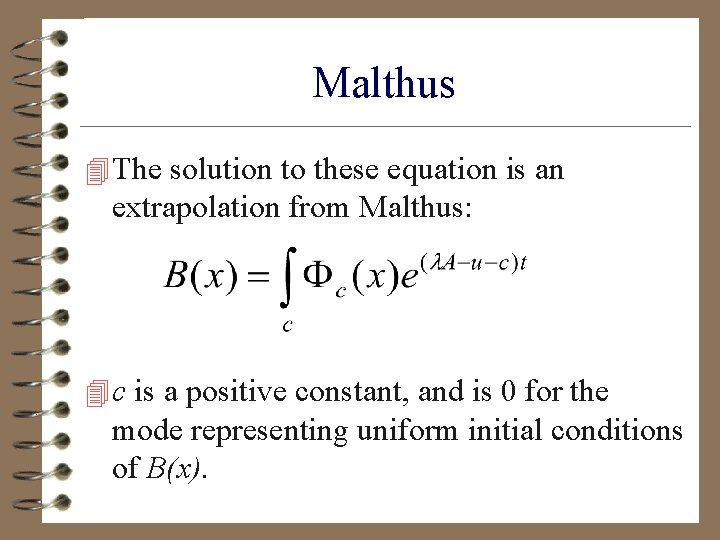Malthus 4 The solution to these equation is an extrapolation from Malthus: 4 c is a positive constant, and is 0 for the mode representing uniform initial conditions of B(x).Naïve PDE II 4 The fastest growing mode has an exponent of: - 4 If - >0, the population will grow until saturated by non linear terms, while if - <0 the population will exponentially decrease to zero (since B is discrete).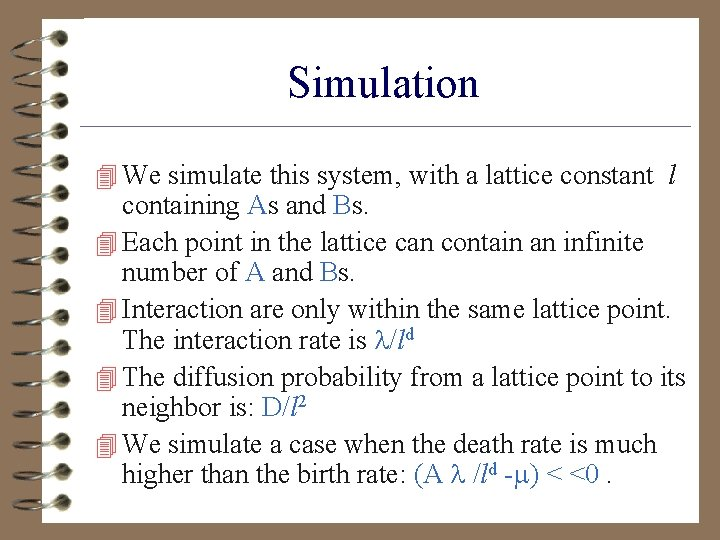Simulation 4 We simulate this system, with a lattice constant l containing As and Bs. 4 Each point in the lattice can contain an infinite number of A and Bs. 4 Interaction are only within the same lattice point. The interaction rate is /ld 4 The diffusion probability from a lattice point to its neighbor is: D/l 2 4 We simulate a case when the death rate is much higher than the birth rate: (A /ld - ) < <0.Simulation Results 4 Even for a very high death rate the total population increases indefinitely (A= 0. 1, /ld =2, =1, A =-0. 8).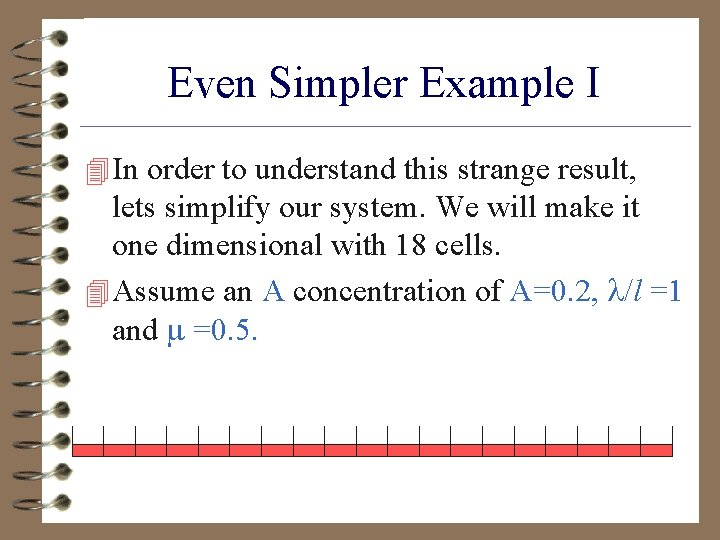Even Simpler Example I 4 In order to understand this strange result, lets simplify our system. We will make it one dimensional with 18 cells. 4 Assume an A concentration of A=0. 2, /l =1 and =0. 5.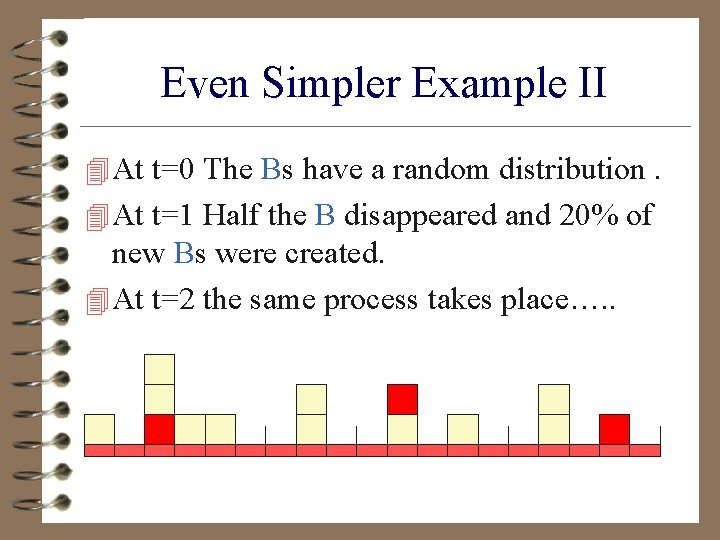Even Simpler Example II 4 At t=0 The Bs have a random distribution. 4 At t=1 Half the B disappeared and 20% of new Bs were created. 4 At t=2 the same process takes place…. .Even Simpler Example III 4 But this is not the social reality, the As are discrete entities. 4 At places where there are no As all the Bs will disappear. 4 At places where at least one A exists the Bs will prosper.Basic Idea 4 This is the result of the combination of an exponential growth and a linear cut-off 4 In an inhomogeneous situation, regions where the local birth rate is lower than the death rate, the total population will simply shrink to zero. 4 In regions where the local birth rate is higher than the death rate the total population will increase to infinity. 4 After a finite time, only regions with a high B population will influence the average B population.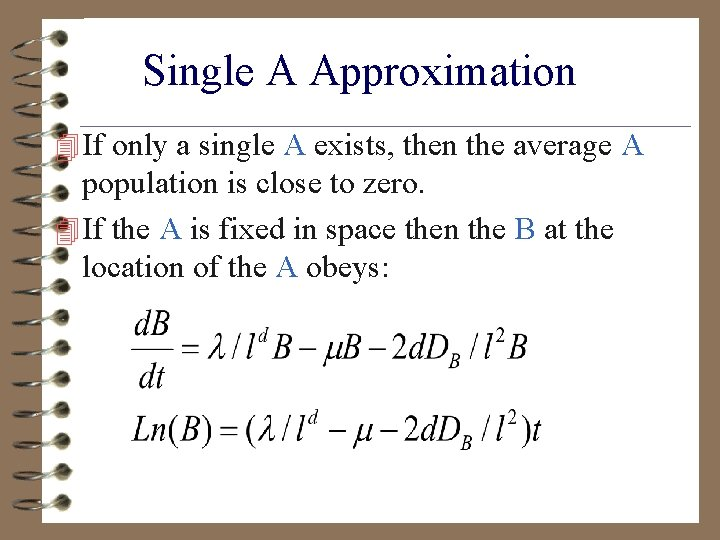Single A Approximation 4 If only a single A exists, then the average A population is close to zero. 4 If the A is fixed in space then the B at the location of the A obeys:But, What About Diffusion? ? ? 4 After a some time the A will diffuse, and all this story will not work any more. 4 Yes!, but the B also diffuses, and by the time the A diffuses there will already be Bs at the new position for the island to keep growing. 4 In every jump the A performs it lands in a region occupied by Bs, but with a lower concentration then at the center of the island.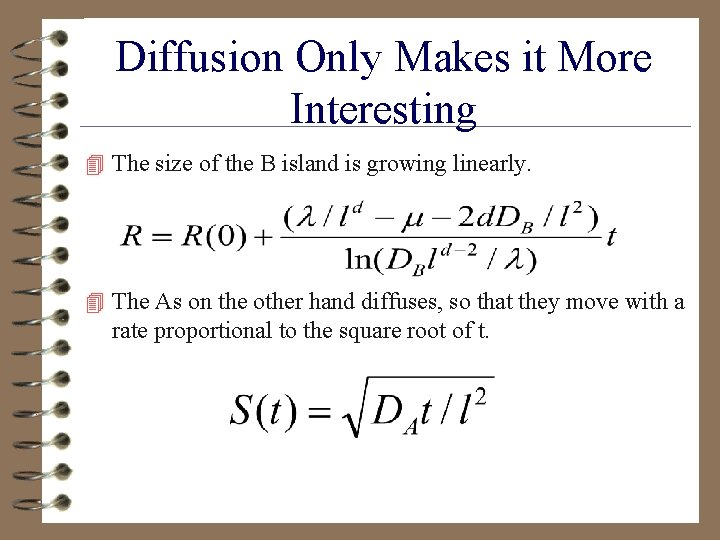Diffusion Only Makes it More Interesting 4 The size of the B island is growing linearly. 4 The As on the other hand diffuses, so that they move with a rate proportional to the square root of t.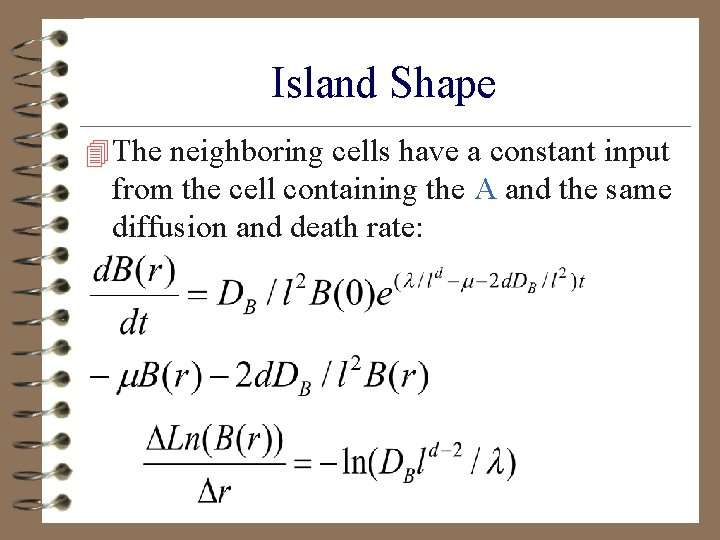Island Shape 4 The neighboring cells have a constant input from the cell containing the A and the same diffusion and death rate:A jumps 4 When A jumps to a neighboring cell, the Ln of the B population at the A position decreases by: 4 The population at the center of the island is thus:Emerging Island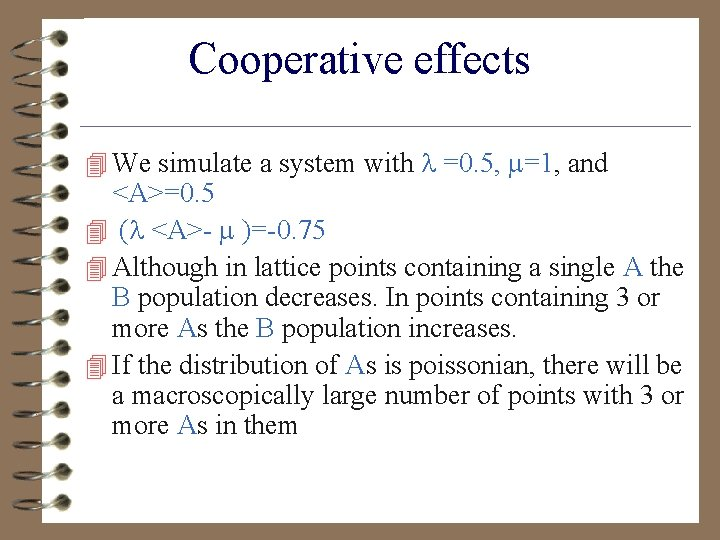Cooperative effects 4 We simulate a system with =0. 5, =1, and <A>=0. 5 4 ( <A>- )=-0. 75 4 Although in lattice points containing a single A the B population decreases. In points containing 3 or more As the B population increases. 4 If the distribution of As is poissonian, there will be a macroscopically large number of points with 3 or more As in them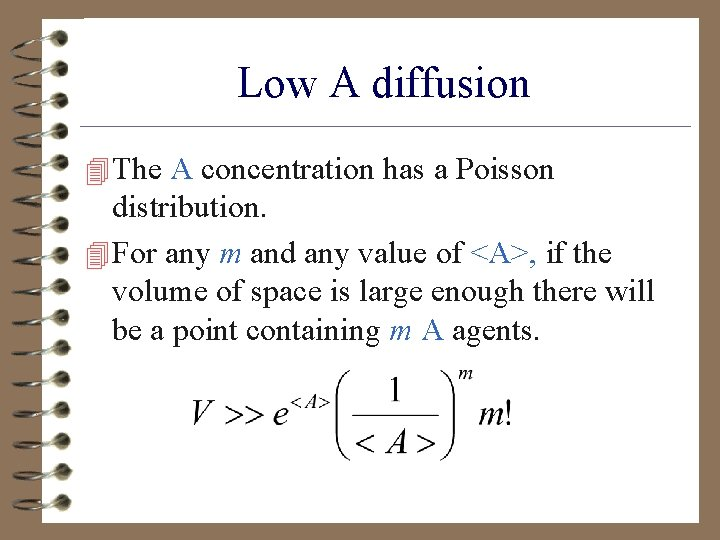Low A diffusion 4 The A concentration has a Poisson distribution. 4 For any m and any value of <A>, if the volume of space is large enough there will be a point containing m A agents.Low A diffusion 4 For any value of , and DB, there is a value m that will obey: 4 The B population will grow precisely in these points. 4 In other words, in the low A diffusion regime the B population grows following the maximum of A and not its average.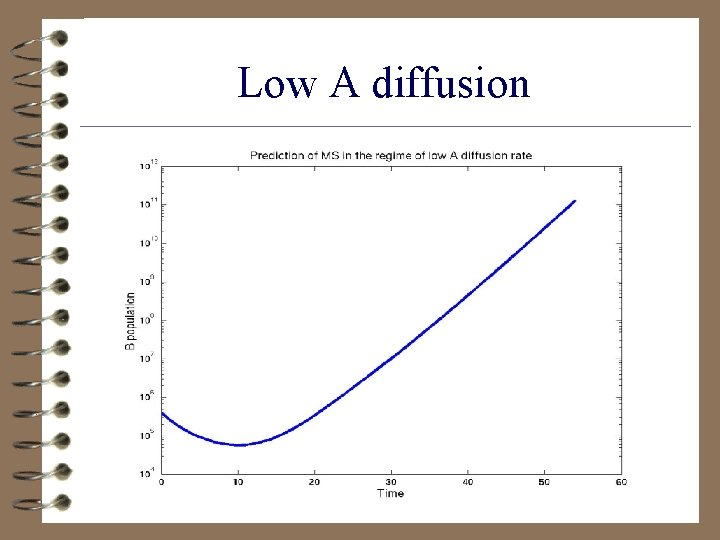Low A diffusionMaster Equation 4 The situation of the system can be described using a master equation for the probability to have A 1 As and B 1 Bs in the first cell… P(A 1 B 1. . ANBN). 4 The master equation for a single point on the lattice is:Translation to quantum formalism 4 In order to solve this equation, we replace the classical probability function by a quantum wave function: 4 >= Pnm/n!/m!(a†)n(b †)m|0>. 4 We define creation and destruction of A and Bs using the quantum creation and annihilation operators: a, a†, b, b†. 4 Adding a new A particle is simply: a†| >, and destroying a particle is: a| >.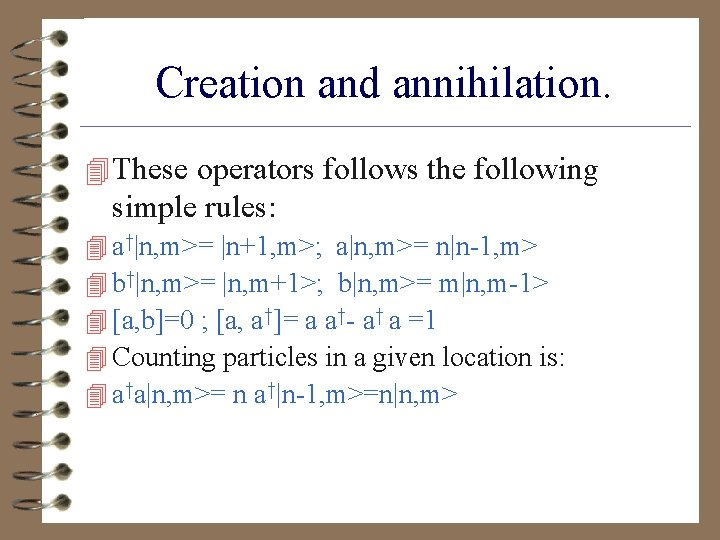Creation and annihilation. 4 These operators follows the following simple rules: 4 a†|n, m>= |n+1, m>; a|n, m>= n|n-1, m> 4 b†|n, m>= |n, m+1>; b|n, m>= m|n, m-1> 4 [a, b]=0 ; [a, a†]= a a†- a† a =1 4 Counting particles in a given location is: 4 a†a|n, m>= n a†|n-1, m>=n|n, m>Hamiltonian 4 We replace the Hamiltonian with a quantum Hamiltonian, and obtain a non imaginary schroedinger equation. 4 ’=H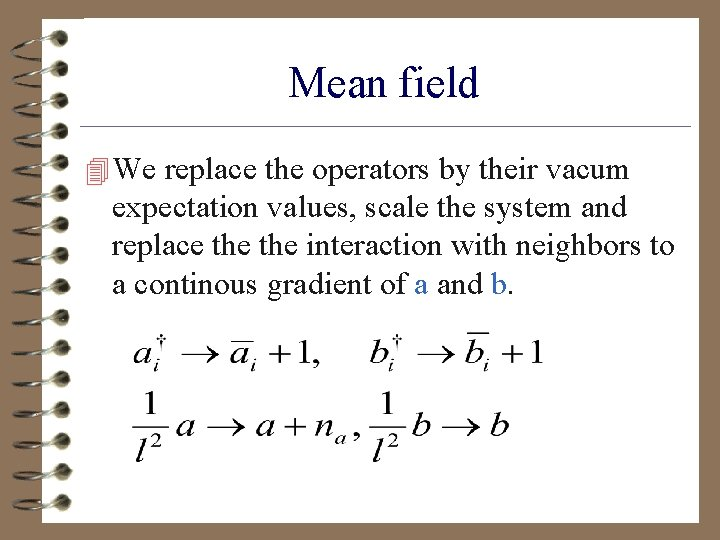Mean field 4 We replace the operators by their vacum expectation values, scale the system and replace the interaction with neighbors to a continous gradient of a and b.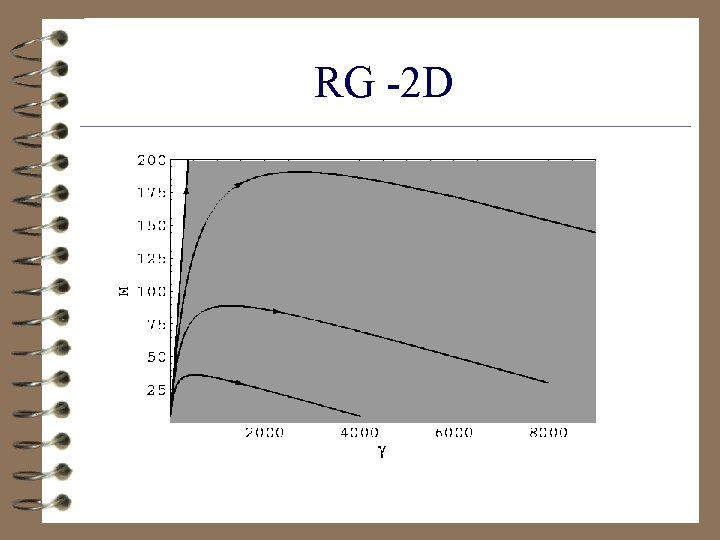RG -2 DRG 3 D 4 In 3 D on the other hand there is a phase transition, and in some of phase space the ODEs are precise.Local Competition 4 The Bs may compete over a local resource (food, space , light…). This local competition is limited to Bs living on the same lattice site. 4 The local competition will mot change the overall dynamics, but it will limit the size and total population of each B island. 4 Any first order interaction can be described in the form of proliferation and death, while any second order mechanism can be described as a competition mechanism.Simulation of competition 4Global Competition 4 The B agents may also compete over a global resource. This happens if the radius in which B compete is larger then the interaction scale between Bs. 4 For example cells competing for a resource in the blood, animals competing over water, plankton competing over oxygen. 4 Large scale competition is described as an interaction with the total population over some scale The competition reaction is : 4 B+<B>-><B>The world company 4 When the A diffusion rate is low only one island of Bs is created around the maximal A concentration. The high B population in this island will inhibit the creation of any other B island. 4 When the As diffuse fast, a number of large B islands are created. These islands look for food (High A concentration ). They can split, merge or die. 4 The life-span of these islands is much larger than the lifespan of a single B. 4 These emerging islands will lead to the creation of intermittent fluctuations in the total B population.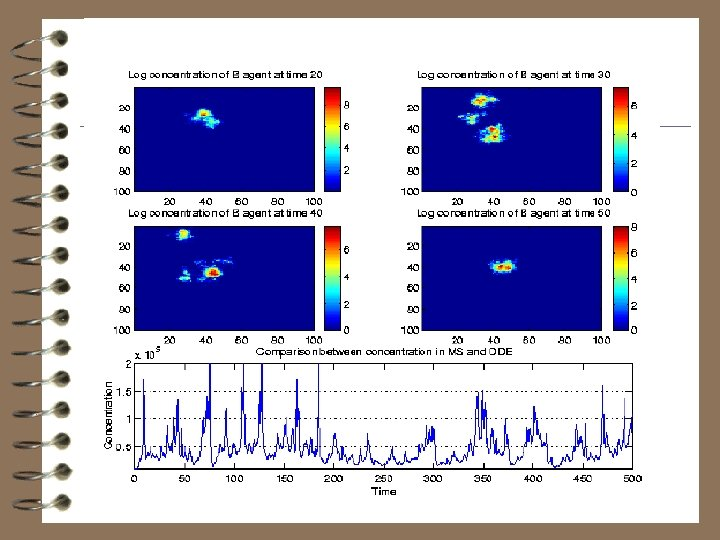Predator prey systems 4 We have shown that the classical PDE treatment of a ver simple autocatalytic systems is wrong. 4 One of the reasons for the large difference is the high correlation between a and b fluctuations. 4 We will show that PDE fail in system with anti-correlation terms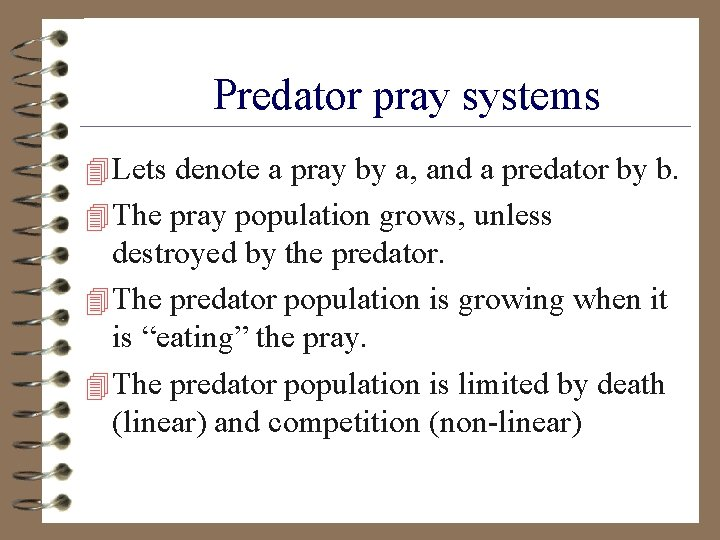Predator pray systems 4 Lets denote a pray by a, and a predator by b. 4 The pray population grows, unless destroyed by the predator. 4 The predator population is growing when it is “eating” the pray. 4 The predator population is limited by death (linear) and competition (non-linear)Ecological niches 4 The equations describing the system are: 4 These equations have 2 fix points: (0, 0) and 4 ([ / + ]/ , / ) , but only the non zero fix point is stable. 4 This is the origin of the ecological niche concept.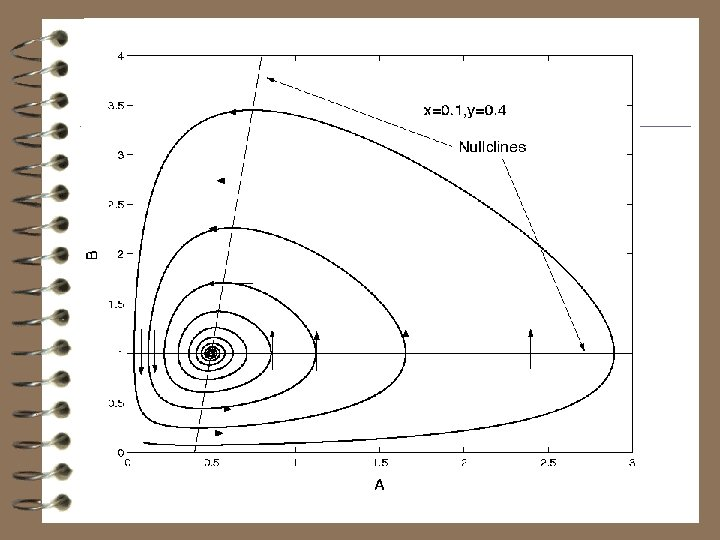Infection Dynamics-ODE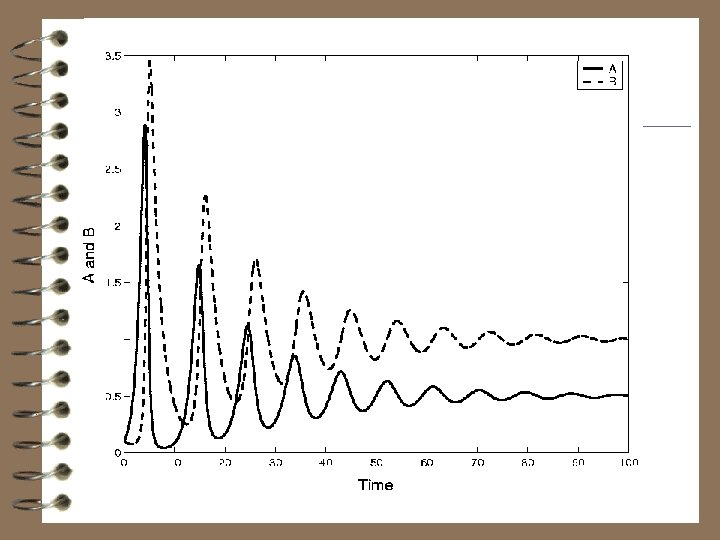Infection Dynamics-ODE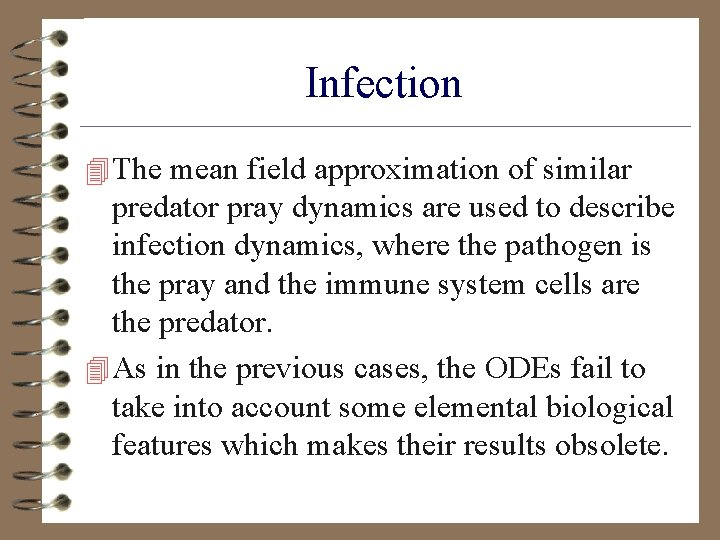Infection 4 The mean field approximation of similar predator pray dynamics are used to describe infection dynamics, where the pathogen is the pray and the immune system cells are the predator. 4 As in the previous cases, the ODEs fail to take into account some elemental biological features which makes their results obsolete.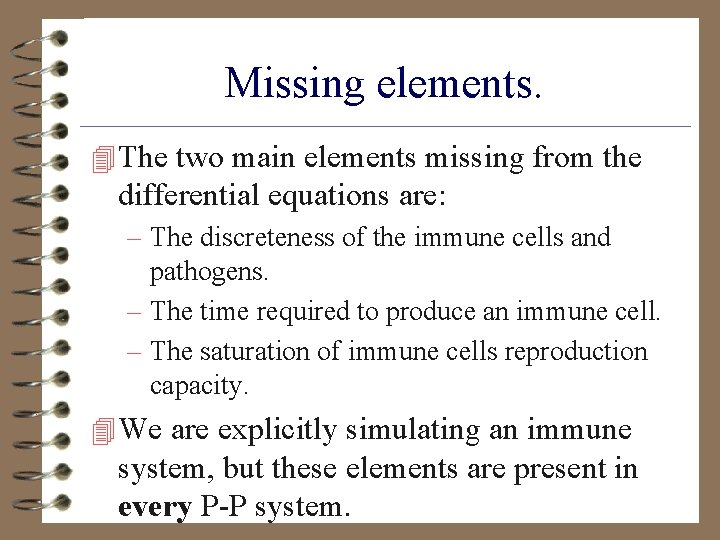Missing elements. 4 The two main elements missing from the differential equations are: – The discreteness of the immune cells and pathogens. – The time required to produce an immune cell. – The saturation of immune cells reproduction capacity. 4 We are explicitly simulating an immune system, but these elements are present in every P-P system.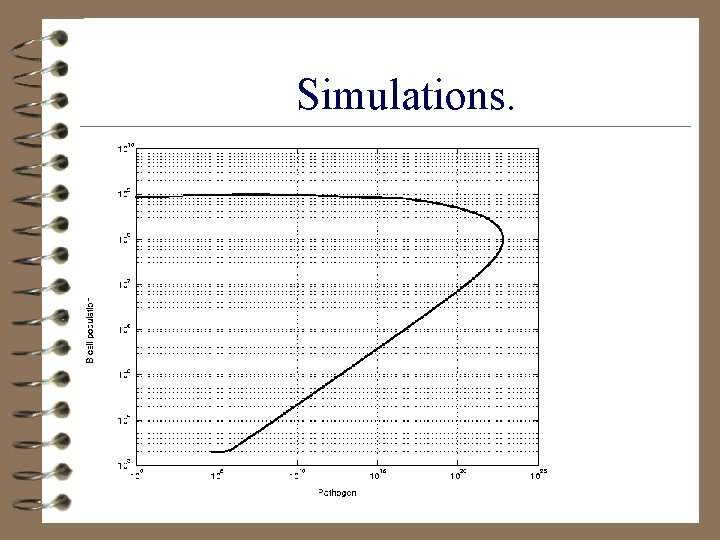Simulations.Spatial distribution. 4 The previous result can be obtained either from a simulation where every point in space have the same random initial distribution, or from a SDE. 4 One can ask what happens if the pathogen is presented in a single point of space.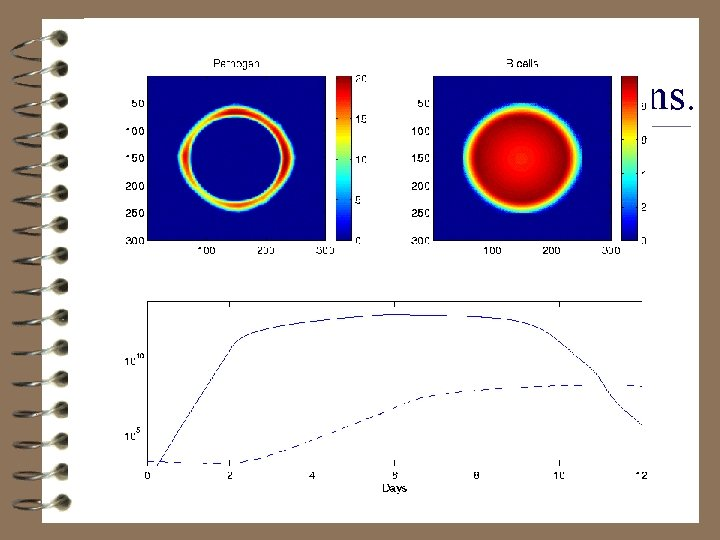Infection Dynamics Simulations.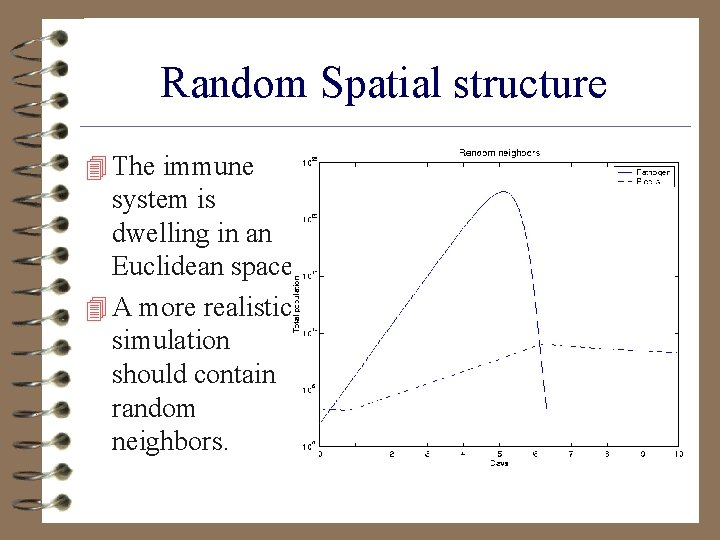Random Spatial structure 4 The immune system is dwelling in an Euclidean space. 4 A more realistic simulation should contain random neighbors.Global destruction. 4 An even more interesting dynamics can take place if the predator has a preying range much larger than the prey diffusion radius. 4 This situation is very frequent. For example lions and tigers have a very wide preying range compared to the grazing range of zebras or antilopes.Infection Dynamics Simulations.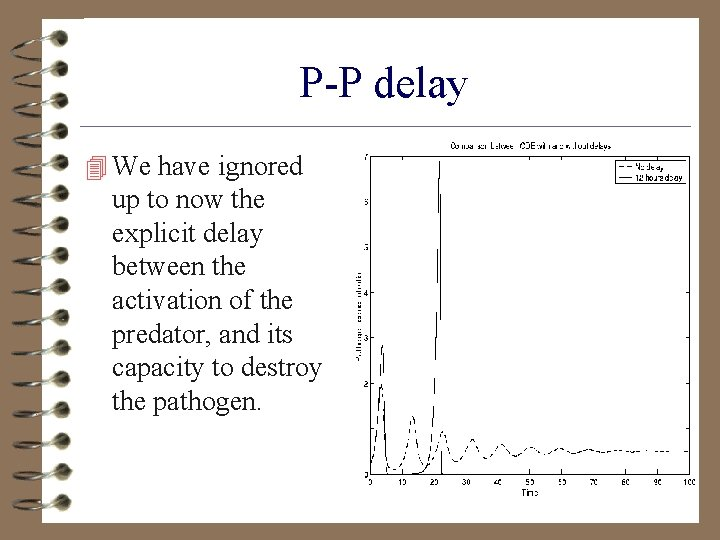P-P delay 4 We have ignored up to now the explicit delay between the activation of the predator, and its capacity to destroy the pathogen.Summary 4 ODEs fail completely in describing autocatalytic systems. 4 The total population of an agent with a lower proliferation rate than death will increase, in contradiction with the homogenous description. 4 The chance of survival are much more important in 2 D than in 3 D. 4 Very simple dynamics can create emerging objects with a long lifespan. In our case these objects are islands of high B concentration around regions of high A concentration.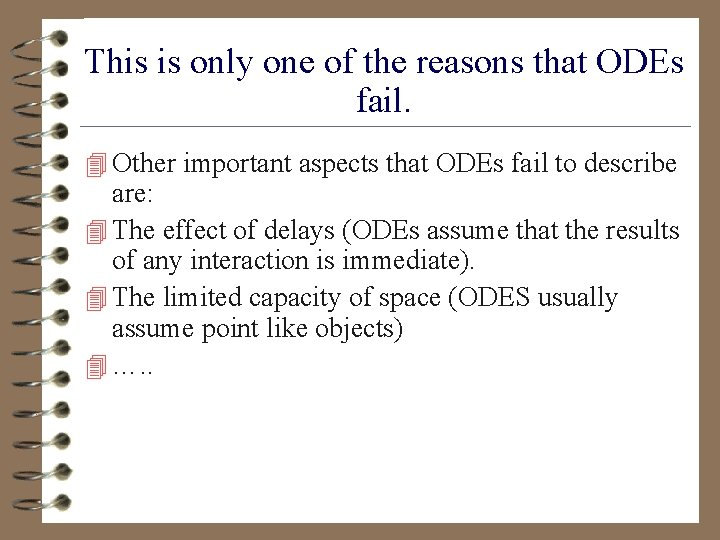This is only one of the reasons that ODEs fail. 4 Other important aspects that ODEs fail to describe are: 4 The effect of delays (ODEs assume that the results of any interaction is immediate). 4 The limited capacity of space (ODES usually assume point like objects) 4 …. .